# Test: Calculus- 1

## 10 Questions MCQ Test GATE Electrical Engineering (EE) 2023 Mock Test Series | Test: Calculus- 1

Description
Attempt Test: Calculus- 1 | 10 questions in 30 minutes | Mock test for GATE preparation | Free important questions MCQ to study GATE Electrical Engineering (EE) 2023 Mock Test Series for GATE Exam | Download free PDF with solutions
QUESTION: 1

### Consider the function y = | x | in the interval [-1, 1], In this interval, the function is

Solution:

The function y = | x | in the interval [-1, 1 ] is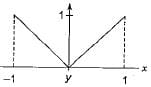| x | is continuous and differentiable every where except at x = 0, where it is continuous but not differentiable.
Since [-1, 1] contains 0, in this interval it is continuous but not differentiable.

QUESTION: 2

###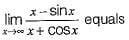Solution: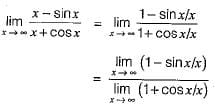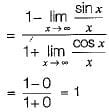QUESTION: 3

### What is the value of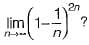Solution: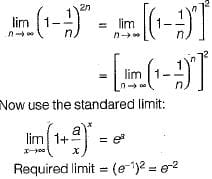QUESTION: 4

Which one of the following functions is continuous at x = 3?

Solution: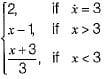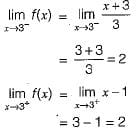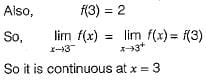QUESTION: 5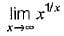is

Solution: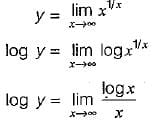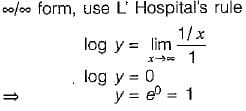QUESTION: 6

Evaluate the limit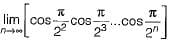Solution: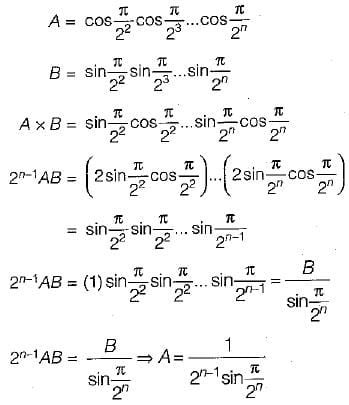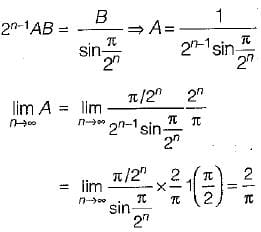QUESTION: 7

The maximum value of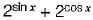Solution: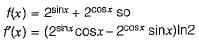to find the maximum it is enough to consider for [ ]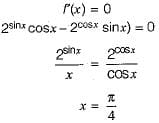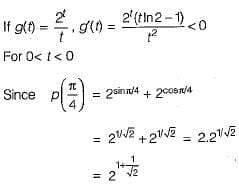QUESTION: 8

A polynomial p(x) is such that p(0) = 5, p(1) = 4, p(2) = 9 and p(3) = 20. The minimum degree it can have is

Solution:

Let, P(x) is of degree 1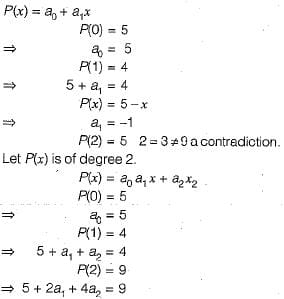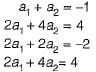Subtract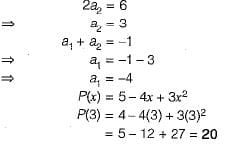QUESTION: 9

The formula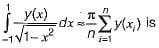Solution:

This is Gauss-Chebyshev formula.

QUESTION: 10

Which of the methods is used for finding solutions of differential equations?

Solution:

Euler method is used for solution of differential equation.Use Code STAYHOME200 and get INR 200 additional OFF Use Coupon Code